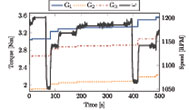Stanton T. Cady with adviser A. Domínguez-García

The focus of this work is to develop and implement distributed algorithms that can be utilized to regulate electrical frequency in a small-footprint power system. We rely on a local controller connected to synchronous generators that are capable of performing simple computations and exchanging information wirelessly with other local controllers of nearby generators. We utilize an iterative algorithm that depends on a pair of states maintained by each local controller. These are updated at each iteration to be a linear combination of the local states and the states of neighboring generators. To account for physical limitations and ratings of the generators, the algorithm’s initial values and final solution are chosen to be a function of the lower and upper capacity constraints.Figure 32: Generator torque and system speed following a load increase.

The 6-bus small-footprint power system illustrated in Figure 31 is implemented in the lab using three synchronous generators rated at 2-hp and 230 Vll. An Arduino microcontroller outfitted with an XBee wireless transceiver which implements the distributed algorithm is connected to the prime mover of each generator. Following a change in the load, one of the local generator controllers measures the frequency error and determines the amount of torque that should be added or removed from the system in order to return the frequency to the desired value. Once computed, the local controllers begin executing the distributed algorithm which serves to disperse the torque error among all of the system generators. Figure 32 illustrates the torque supplied by each of the three system generators as well as the mechanical speed of the generators following load increases at about 75 and 400 seconds. As Figure 32 shows, the algorithm effectively returns the frequency close to the desired value of 1200 RPM. This research is supported by the Power System Engineering Research Center.

This research is funded by the Grainger Center for Electric Machinery and Electromechanics.